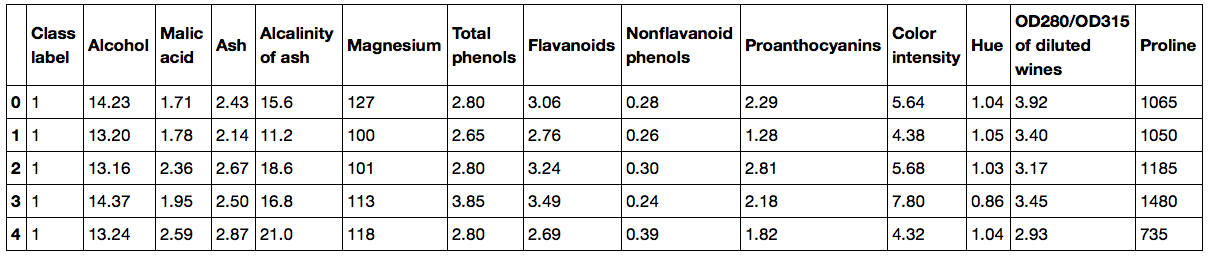All Courses

# How to Partitioning a dataset in training and test sets using Scikit-learn?

##### By Rama, 2 years ago

Partitioning the Wine dataset is open-source dataset that is available from the UCI machine learning repository into train and test dataset.

Tain set
Test set
Scikit-learn
##### Albert

Using the pandas library, we will directly read in the open source Wine dataset from the UCI machine learning repository:

```import pandas as pd
import numpy as np

df_wine.columns = ['Class label', 'Alcohol', 'Malic acid', 'Ash','Alcalinity of ash', 'Magnesium','Total phenols', 'Flavanoids',
'Nonflavanoid phenols','Proanthocyanins','Color intensity', 'Hue','OD280/OD315 of diluted wines','Proline']

print('Class labels', np.unique(df_wine['Class label']))
```

```Class labels [1 2 3]
```

```df_wine.head()
```A convenient way to randomly partition this dataset into a separate test and training dataset is to use the train_test_split function from scikit-learn's cross_validation submodule

```>>> from sklearn.cross_validation import train_test_split
>>> X, y = df_wine.iloc[:, 1:].values, df_wine.iloc[:, 0].values
>>> X_train, X_test, y_train, y_test = train_test_split(X, y, test_size=0.3, random_state=0)
```

First, we assigned the NumPy array representation of feature columns 1-13 to the variable X , and we assigned the class labels from the first column to the variable y . Then, we used the train_test_split function to randomly split X and y into

separate training and test datasets. By setting test_size=0.3 we assigned 30 percent of the wine samples to X_test and y_test , and the remaining 70 percent of the samples were assigned to X_train and y_train , respectively.

#### How To Land a Job in Data Science?Feb 9th (7:00 PM) 184 Registered
More webinars

#### Related Discussions

Running random forest algorithm with one variable

View More